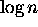Tom Kelliher, CS 315

Mar. 1, 999

### Announcements

Collect homework.

### Coming Up

Parallel prefix computations.

1. In general, the sum of two n-bit numbers results in an n+1-bit sum.

2. The problem reduces to computing the carries.

3. For each bit position we determine if it would
1. Propagate a carry (p).

2. Generate a carry (g).

3. Stop a carry (s).

What's the ``truth table'' for this.

4. Example: 0xf53a and 0xc796.

5. The computation is carried out in a binary tree with addend, augend bits transmitted to the leaves.

Dimensions of the tree?

6. Steps of the computations:
1. Step 1: compute p, g, s in the leaves.

2.ascending steps:
1. Receive inputs from children, who ``erase'' their values.

2. Pass value received from right child to left child.

3. Compute own value to pass to parent. What is the computation?

3. Special case for root:
1. Its value becomes the carry-out (or additional sum bit). Output this value.

2. Set value to s.

4.descending steps:

2. Keep first non-p value received (including the value received by a left child from a right child).

7. When finished, all leaves are labeled either s or g. Which is a carry-in of 0 and which is 1?

8. We know no leaf will be ``stuck'' with a p. Why?

9. The carry-in for a leaf is the left-most non-p value to the right of the leaf, as originally computed by the leaves.

Prove that the algorithm stores this carry in each leaf.

Thomas P. Kelliher
Mon Mar 1 09:04:43 EST 1999
Tom Kelliher